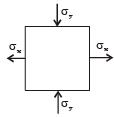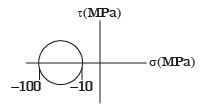Courses

# GATE Past Year Questions: Mohr's Circle Notes | EduRev

## Mechanical Engineering : GATE Past Year Questions: Mohr's Circle Notes | EduRev

The document GATE Past Year Questions: Mohr's Circle Notes | EduRev is a part of the Mechanical Engineering Course Strength of Materials (SOM).
All you need of Mechanical Engineering at this link: Mechanical Engineering

Try yourself:The state of stress at a point P in a two dimensional loading is such that the Mohr's circle is a point located at 175 MPa on the positive normal stress axis.
The maximum and minimum principal stresses respectively from the Mohr's circle are



Try yourself:The state of stress at a point P in a two dimensional loading is such that the Mohr's circle is a point located at 175 MPa on the positive normal stress axis.
The directions of maximum and minimum principal stresses at the point P from the Mohr's circle are



Try yourself:The figure shows the state of stress at a certain point in a stressed body. The magnitudes of normal stresses in the x and y directions are 100 MPa and 20 MPa respectively. The radius of Mohr's stress circle representing this state of stress isTry yourself:The Mohr's circle of plane stress for a point in a body is shown. The design is to be done on the basis of the maximum shear stress theory for yielding. Then, yielding will just begin if the designer chooses a ductile material whose yield strength isTry yourself:A two dimensional fluid element rotates like a rigid body. At a point within the element, the pressure is 1 unit. Radius of the Mohr's circle, characterizing the state at that point, is



Try yourself:The state of stress at a point under plane stress condition is
σxx = 40 MPa, σyy = 100 MPa and σxy  =40 MPa
The radius of Mohr's circle representing the given state of stress in MPa is



Offer running on EduRev: Apply code STAYHOME200 to get INR 200 off on our premium plan EduRev Infinity!

## Strength of Materials (SOM)

35 videos|33 docs|48 tests

,

,

,

,

,

,

,

,

,

,

,

,

,

,

,

,

,

,

,

,

,

;